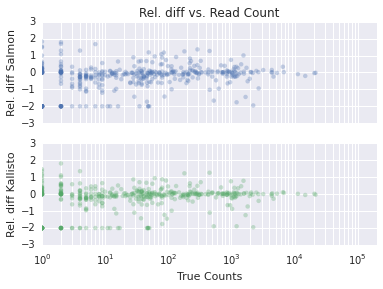This is a (slightly modified) reproduction of Simon's quantification comparison analysis, but in an iPython Notebook

In :
%matplotlib inline
import pandas as pd
import seaborn as sns
import matplotlib.pyplot as plt
import numpy as np


Some constants and useful variables

In :
MEAN_FL = 250
small = 1e-2 # for log things (use same value as Harold)


Some basic functions

In :
# Truncate values in colname < val to 0
def filterExpression(df, colname="TPM", val=0.01):
df[colname][df[colname] < val] = 0

In :
# Join a list of DataFrames together, merging on the index and renaming conflicting columns
def joinFrames(frames):
current = frames
for i, frame in enumerate(frames[1:], 2):
current = current.join(frame, rsuffix='{}'.format(i))
return current


Load all of the simulation files for Salmon & Kallisto

In :
salmon_files = ["sim_test_salmon_{}/quant.sf".format(i) for i in xrange(1,11)]
salmonDataFrames = []
for fn in salmon_files:
# Rename #Name => Name
df = df.rename(columns={'# Name': 'Name'})
df = df.set_index('Name')
salmonDataFrames.append(df)

In :
kallisto_files = ["sim_test_kallisto_bs/bs_abundance_{}.txt".format(i) for i in xrange(10)]
kallistoDataFrames = []
for fn in kallisto_files:
# Rename #Name => Name
df = df.rename(columns={'target_id' : 'Name', 'length' : 'Length', 'est_counts' : 'NumReads', 'tpm' : 'TPM'})
df = df.set_index('Name')
kallistoDataFrames.append(df)


For all the simulations for each method, merge the results into a single data frame for easier analysis

In :
mergedSalmon = joinFrames(salmonDataFrames)
mergedSalmon["TPMAvg"] = mergedSalmon[["TPM"] + ["TPM{}".format(i) for i in xrange(2,11)]].mean(axis=1)

In :
mergedKallisto = joinFrames(kallistoDataFrames)
mergedKallisto["TPMAvg"] = mergedKallisto[["TPM"] + ["TPM{}".format(i) for i in xrange(2,11)]].mean(axis=1)


Load the ground truth data, the results of simulation 1 for Salmon, and the results on the full data for Kallisto

In :
salmon = pd.read_csv('sim_test_salmon_1/quant.sf', sep='\t', skiprows=11)
salmon = salmon.rename(columns={'# Name': 'Name'})
salmon = salmon.set_index('Name')

In :
kallisto = pd.read_csv('sim_test_kallisto/abundance.txt', sep='\t')
kallisto = kallisto.rename(columns={'target_id' : 'Name', 'length' : 'Length', 'est_counts' : 'NumReads', 'tpm' : 'TPM'})
kallisto = kallisto.set_index('Name')


Apparently there are some transcripts that are in the simulation, but they are not in the ground truth file ...

This code drops expressions for ground truth transcripts that were not included in the simulation, and sets "ground truth" values of 0 for those transcripts (mentioned above) that are in the simulation but not the expression ground truth file.

The original TPMs were on a larger dataset, so re-normalize them (but do it according to rounded counts --- thanks for pointing this out Harold!)

In :
# Load the ground truth files
# Rename #Name => Name
truth = truth.rename(columns={'# Name':'Name'})
truth = truth.set_index('Name')

# There were transcripts that *were* selected for the simulation, but they *were not* in the
truth = truth.loc[kallisto.index]
truth.fillna(0, inplace=True)
# Since we filled all NaN's with 0, replace the length here
truth["Length"] = kallisto["Length"]
# Round the counts

# The transcript fraction
truth["EffectiveLen"] = truth["Length"] - MEAN_FL
# Don't allow a negative effective length
truth["EffectiveLen"][truth["EffectiveLen"] < 0] = 0

# Any reads from transcripts with no effective length

# From the true transcript fractions, compute the true TPM with effective lengths
transcriptFracs = (roundedCounts / truth["EffectiveLen"])
transcriptFracs[transcriptFracs == np.inf] = 0
trueTPMEffectiveLen = 1000000.0 * (transcriptFracs / sum(transcriptFracs.values))
truth["TPM_EL_truth"] = trueTPMEffectiveLen

# From the true transcript fractions, compute the true TPM with actual lengths
transcriptFracs = (roundedCounts / truth["Length"])
transcriptFracs[transcriptFracs == np.inf] = 0
trueTPMRealLen = 1000000.0 * (transcriptFracs / sum(transcriptFracs.values))
truth["TPM_RL_truth"] = trueTPMRealLen

# Now, let the true counts be our rounded counts

Number of 'Strange' reads = 0


Create some data frames for Salmon and Kallisto that contain the ground truth values as columns

In :
truthSalmon = truth.join(mergedSalmon, lsuffix='_truth', rsuffix='_salmon')

In :
truthKallisto = truth.join(kallisto, lsuffix='_truth', rsuffix='_kallisto')


## Some simple correlations to start off¶

In :
# correlation with the average salmon TPM
print("TPM correlation Salmon (Avg) vs. Truth (RealLen) = {}".format(truthSalmon.corr(method='spearman')["TPM_RL_truth"]["TPMAvg"]))
print("TPM correlation Salmon (Avg) vs. Truth (EffLen) = {}".format(truthSalmon.corr(method='spearman')["TPM_EL_truth"]["TPMAvg"]))

TPM correlation Salmon (Avg) vs. Truth (RealLen) = 0.83495978804
TPM correlation Salmon (Avg) vs. Truth (EffLen) = 0.834077786529

In :
# and with the first replicate
print("TPM correlation Salmon (Sim1) vs. Truth (RealLen) = {}".format(truthSalmon.corr(method='spearman')["TPM_RL_truth"]["TPM_salmon"]))
print("TPM correlation Salmon (Sim1) vs. Truth (EffLen) = {}".format(truthSalmon.corr(method='spearman')["TPM_EL_truth"]["TPM_salmon"]))

TPM correlation Salmon (Sim1) vs. Truth (RealLen) = 0.835845618235
TPM correlation Salmon (Sim1) vs. Truth (EffLen) = 0.834965112553

In :
# correlation with Kallisto
print("TPM correlation Kallisto vs. Truth (RealLen) = {}".format(truthKallisto.corr(method='spearman')["TPM_RL_truth"]["TPM_kallisto"]))
print("TPM correlation Kallisto vs. Truth (EffLen) = {}".format(truthKallisto.corr(method='spearman')["TPM_EL_truth"]["TPM_kallisto"]))

TPM correlation Kallisto vs. Truth (RealLen) = 0.83485831708
TPM correlation Kallisto vs. Truth (EffLen) = 0.835781563454


## Coefficient of Variation Plots ¶

As noted by Harold, these don't really have the same meaning between Salmon and Kallisto, but I'm reproducing them here since they were in the original analysis.

In :
p = sns.regplot(mergedSalmon["NumReadsAvg"], mergedSalmon["NumReadsStdDev"] / mergedSalmon["NumReadsAvg"], fit_reg=False)
_ = p.set_ylabel("CV")
_ = p.set_title("Variability Salmon")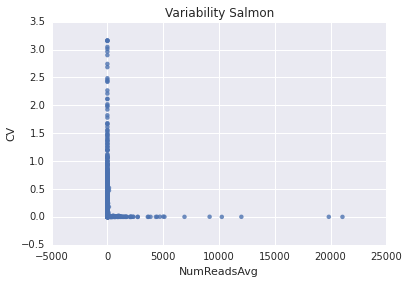In :
p = sns.regplot(mergedKallisto["NumReadsAvg"], mergedKallisto["NumReadsStdDev"] / mergedKallisto["NumReadsAvg"], fit_reg=False)
_ = p.set_ylabel("CV")
_ = p.set_title("Variability Kallisto")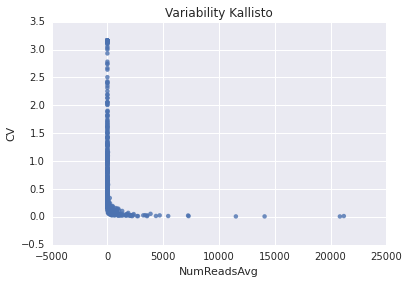## TPM correlation plots¶

In :
p = sns.regplot(np.log(truthSalmon["TPM_RL_truth"].values+1), np.log(truthSalmon["TPM_salmon"]+1), truthSalmon)
p.set_xlabel("true TPM (RealLen)")
p.set_ylabel("Salmon (Sim1) TPM")
p.set_title("Salmon TPM (Sim1) correlation")
sr = truthSalmon.corr(method='spearman')["TPM_RL_truth"]["TPM_salmon"]
print("Spearman r = {}".format(sr))

Spearman r = 0.835845618235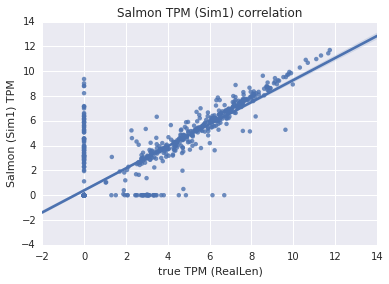In :
p = sns.regplot(np.log(truthSalmon["TPM_EL_truth"].values+1), np.log(truthSalmon["TPM_salmon"]+1), truthSalmon)
p.set_xlabel("true TPM (EffLen)")
p.set_ylabel("Salmon (Sim1) TPM")
p.set_title("Salmon TPM (Sim1) correlation")
sr = truthSalmon.corr(method='spearman')["TPM_EL_truth"]["TPM_salmon"]
print("Spearman r = {}".format(sr))

Spearman r = 0.834965112553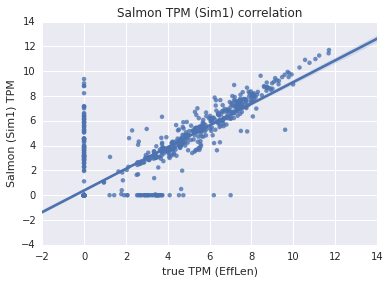In :
p = sns.regplot(np.log(truthKallisto["TPM_RL_truth"].values+1), np.log(truthKallisto["TPM_kallisto"].values+1), truthKallisto)
p.set_xlabel("true TPM (RealLen)")
p.set_ylabel("Kallisto (FullData) TPM")
p.set_title("Kallisto TPM correlation")
sr = truthKallisto.corr(method='spearman')["TPM_RL_truth"]["TPM_kallisto"]
print("Spearman r = {}".format(sr))

Spearman r = 0.83485831708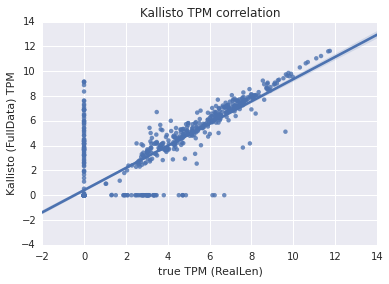In :
p = sns.regplot(np.log(truthKallisto["TPM_EL_truth"].values+1), np.log(truthKallisto["TPM_kallisto"].values+1), truthKallisto)
p.set_xlabel("true TPM (EffLen)")
p.set_ylabel("Kallisto (FullData) TPM")
p.set_title("Kallisto TPM correlation")
sr = truthKallisto.corr(method='spearman')["TPM_EL_truth"]["TPM_kallisto"]
print("Spearman r = {}".format(sr))

Spearman r = 0.835781563454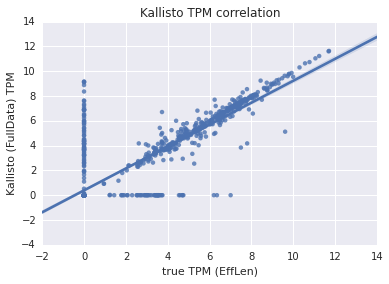## Correlations between numbers of reads¶

This is useful because it "sidesteps" the effective length issue

In :
p = sns.regplot(np.log(truthSalmon["NumReads_truth"].values+1), np.log(truthSalmon["NumReads_salmon"]+1), truthSalmon)
print("Spearman r = {}".format(sr))

Spearman r = 0.834552169711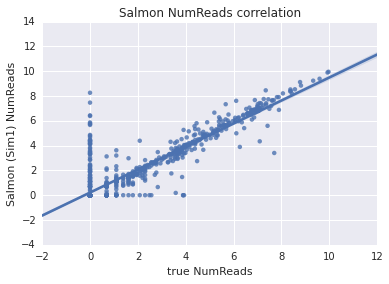In :
p = sns.regplot(np.log(truthSalmon["NumReads_truth"].values+1), np.log(truthKallisto["NumReads_kallisto"]+1), truthKallisto)
print("Spearman r = {}".format(sr))

Spearman r = 0.836134057877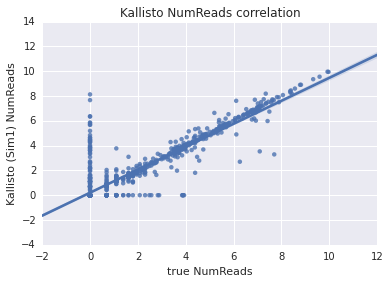## No big changes, but let's see how those correlations looks if we apply some "reasonable" cutoffs¶

In :
# Maintain an un-filtered copy
truthSalmonUnfiltered = truthSalmon.copy()
truthKallistoUnfiltered = truthKallisto.copy()

# No big changes, but let's see how everything looks if we apply some "reasonable" cutoffs
filterExpression(truthSalmon, colname="TPM_salmon", val=0.01)
filterExpression(truthSalmon, colname="TPMAvg", val=0.01)
filterExpression(truthSalmon, colname="TPM_RL_truth", val=0.01)
filterExpression(truthSalmon, colname="TPM_EL_truth", val=0.01)

filterExpression(truthKallisto, colname="TPM_kallisto", val=0.01)
filterExpression(truthKallisto, colname="TPM_RL_truth", val=0.01)
filterExpression(truthKallisto, colname="TPM_EL_truth", val=0.01)


## (Filtered) TPM correlation plots¶

In :
p = sns.regplot(np.log(truthSalmon["TPM_RL_truth"].values+1), np.log(truthSalmon["TPM_salmon"]+1), truthSalmon)
p.set_xlabel("true TPM (RealLen)")
p.set_ylabel("Salmon (Sim1) TPM")
p.set_title("Salmon TPM (Sim1) correlation")
sr = truthSalmon.corr(method='spearman')["TPM_RL_truth"]["TPM_salmon"]
print("Spearman r = {}".format(sr))

Spearman r = 0.84651452142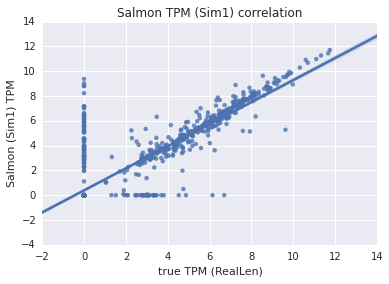In :
p = sns.regplot(np.log(truthSalmon["TPM_EL_truth"].values+1), np.log(truthSalmon["TPM_salmon"]+1), truthSalmon)
p.set_xlabel("true TPM (EffLen)")
p.set_ylabel("Salmon (Sim1) TPM")
p.set_title("Salmon TPM (Sim1) correlation")
sr = truthSalmon.corr(method='spearman')["TPM_EL_truth"]["TPM_salmon"]
print("Spearman r = {}".format(sr))

Spearman r = 0.845740329822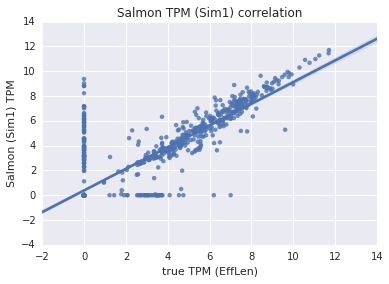In :
p = sns.regplot(np.log(truthKallisto["TPM_RL_truth"].values+1), np.log(truthKallisto["TPM_kallisto"].values+1), truthKallisto)
p.set_xlabel("true TPM (RealLen)")
p.set_ylabel("Kallisto (FullData) TPM")
p.set_title("Kallisto TPM correlation")
sr = truthKallisto.corr(method='spearman')["TPM_RL_truth"]["TPM_kallisto"]
print("Spearman r = {}".format(sr))

Spearman r = 0.833023060229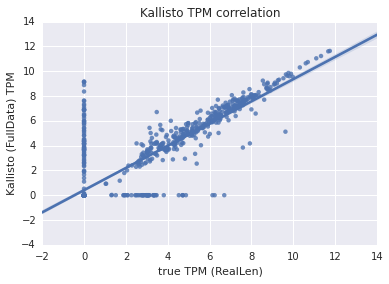In :
p = sns.regplot(np.log(truthKallisto["TPM_EL_truth"].values+1), np.log(truthKallisto["TPM_kallisto"].values+1), truthKallisto)
p.set_xlabel("true TPM (EffLen)")
p.set_ylabel("Kallisto (FullData) TPM")
p.set_title("Kallisto TPM correlation")
sr = truthKallisto.corr(method='spearman')["TPM_EL_truth"]["TPM_kallisto"]
print("Spearman r = {}".format(sr))

Spearman r = 0.834136306562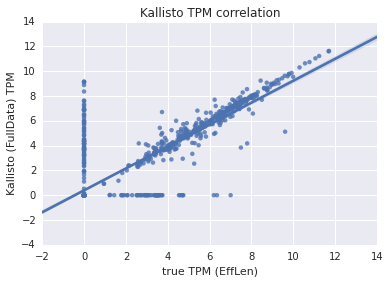## (Filtered) Read Count correlation plots¶

In :
p = sns.regplot(np.log(truthSalmon["NumReads_truth"].values+1), np.log(truthSalmon["NumReads_salmon"]+1), truthSalmon)
print("Spearman r = {}".format(sr))

Spearman r = 0.839419059657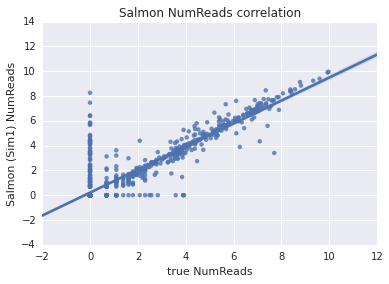In :
p = sns.regplot(np.log(truthSalmon["NumReads_truth"].values+1), np.log(truthKallisto["NumReads_kallisto"]+1), truthKallisto)
print("Spearman r = {}".format(sr))

Spearman r = 0.837290633981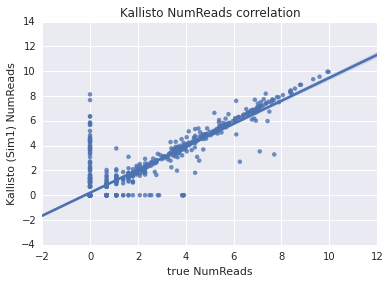In :
def relDiff(c1, c2, DF, verbose=False):
rd = pd.DataFrame(data = {"Name" : DF.index, "relDiff" : np.zeros(len(DF.index))*np.nan})
rd.set_index("Name", inplace=True)
bothZero = DF[(DF[c1] == 0) & (DF[c2] == 0)].index
nonZero = DF.index - bothZero
if (verbose):
print("Zero occurs in both columns {} times".format(len(rd.loc[bothZero])))
print("Nonzero occurs in at least 1 column {} times".format(len(rd.loc[nonZero])))
allRD = 2.0 * ((DF[c1] - DF[c2]) / (DF[c1] + DF[c2]).abs())
assert(len(rd.loc[nonZero]["relDiff"]) == len(allRD[nonZero]))
rd["relDiff"][nonZero] = allRD[nonZero]
rd["relDiff"][bothZero] = 0.0
return rd, nonZero


We use the same definition here as Harold. The relative difference between the predicted expression $x_i$ and the true expression $y_i$ is given as:

$$d_i = \frac{x_i - y_i}{\frac{1}{2}\left|x_i + y_i\right|}$$

Further note: Here, we are dealing with the truncated data. Specifically, this still considers all transcripts, but it treats any TPM < 0.01 as 0 and any read count < 1 as 0. I believe that these are truly the more representative statistics (because, e.g. it normalizes between methods that truncate expression values internally and those that do not --- see below). With the un-truncated estimates, methods that prefer to report a vanishingly small (but non-zero) estimated abundance are heavily penalized under the relative difference metric. For example, imagine a set of transcripts with true expression (TPM) 0 and estimated expression (TPM) of $1 \times 10^{-30}$ --- each of these transcripts would have a relative difference of $2 = \frac{1 \times 10^{-30} - 0}{\frac{1}{2}\left|1 \times 10^{-30} + 0\right|}$. This is (in terms of absolute value), the largest possible relative difference, but, because of the estimated expression value (which is miniscule) it is not particularly meaningful. Let's take a look at how significant of a differerence this can make by looking at some (non-zero) median relative errors:

In :
rdS, nzS = relDiff("TPM_salmon", "TPM_RL_truth", truthSalmonUnfiltered)
medRelDiffSalmonUnfilt = rdS["relDiff"][nzS].median()

X = truthSalmonUnfiltered.copy()
filterExpression(X, colname='TPM_salmon', val=1e-30)

rdS, nzS = relDiff("TPM_salmon", "TPM_RL_truth", X)
medRelDiffSalmonFilt = rdS["relDiff"][nzS].median()

filterExpression(X, colname='TPM_RL_truth', val=1e-30)

rdS, nzS = relDiff("TPM_salmon", "TPM_RL_truth", X)
medRelDiffSalmonFiltBoth = rdS["relDiff"][nzS].median()

print("Median (non-zero) relative differences\n========================================")
print("Unfiltered = {}".format(medRelDiffSalmonUnfilt))
print("Filtered (salmon TPM < 1e-30 => 0.0) = {}".format(medRelDiffSalmonFilt))
print("Filtered (salmon TPM < 1e-30 => 0.0 and true TPM < 1e-30 => 0.0) = {}".format(medRelDiffSalmonFiltBoth))

Median (non-zero) relative differences
========================================
Unfiltered = 0.456184462872
Filtered (salmon TPM < 1e-30 => 0.0) = 0.0453498332992
Filtered (salmon TPM < 1e-30 => 0.0 and true TPM < 1e-30 => 0.0) = 0.0453498332992


As we can see above, the median relative difference of $\sim 0.46$ (relative difference always falls in $\left[-2.0, 2.0\right]$, so this value is seemingly large) is caused by tiny estimated TPMs ($< 1 \times 10^{-30}$) for transcripts with a true TPM that is even smaller (likely $0$). By truncating these tiny TPM values to 0, we obtain a median relative difference of $0.046$ (an order of magnitude smaller) --- this is without any knowledge of the ground truth expression and also without setting those expressions to $0$. If we also truncate to $0$ any tiny true TPM values, the median relative difference remains essentially the same (as expected). Thus, applying such a filter to estimated expression values yields a much more meaningful comparison between methods, especially since some methods already apply a gentle filtering to eliminate such small estimated expression values internally (see e.g. here). Thus, we proceed withe these results below.

# First, we'll look at TPMs again¶

In :
rdK, nzK = relDiff("TPM_kallisto", "TPM_RL_truth", truthKallisto)
medRelDiffKallisto = rdK["relDiff"].median()

rdS, nzS = relDiff("TPM_salmon", "TPM_RL_truth", truthSalmon)
medRelDiffSalmon = rdS["relDiff"].median()
relDiffTPM = pd.DataFrame(rdK).join(pd.DataFrame(rdS), lsuffix='_kallisto', rsuffix='_salmon')

In :
print("Kallisto")
print("===============================")
print("Median relative difference = {}".format(medRelDiffKallisto))
print("Median relative difference (non-zero) = {}".format(rdK["relDiff"][nzK].median()))
print("Mean relative difference = {}".format(rdK["relDiff"].mean()))

print("\n\nSalmon")
print("===============================")
print("Median relative difference = {}".format(medRelDiffSalmon))
print("Median relative difference (non-zero) = {}".format(rdS["relDiff"][nzS].median()))
print("Mean relative difference = {}".format(rdS["relDiff"].mean()))

Kallisto
===============================
Median relative difference = 0.0
Median relative difference (non-zero) = 0.0356676963046
Mean relative difference = 0.0744219582712

Salmon
===============================
Median relative difference = 0.0
Median relative difference (non-zero) = -0.0182341267813
Mean relative difference = 0.042149132981

In :
p = plt.hist(relDiffTPM["relDiff_salmon"].values, bins=100)
_ = plt.axes().set_ylim((0,700))
_ = plt.axes().set_title("Salmon TPM Relative Difference")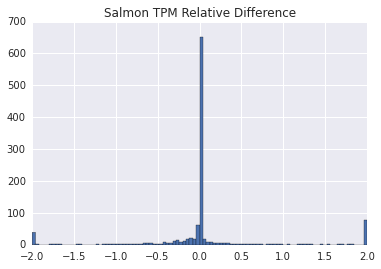In :
p = plt.hist(relDiffTPM["relDiff_kallisto"].values, bins=100)
_ = plt.axes().set_ylim((0,700))
_ = plt.axes().set_title("Kallisto TPM Relative Difference")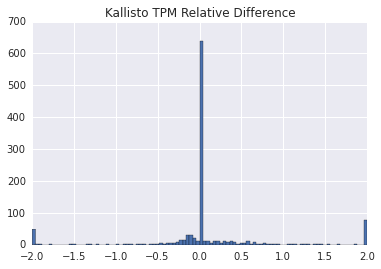In :
f, ax = plt.subplots(2, 1, sharex=True)
p = sns.regplot(truthSalmon["TPM_RL_truth"].values, rdS["relDiff"].values, scatter_kws={'alpha' : 0.3}, fit_reg=False, ax=ax)
p2 = sns.regplot(truthKallisto["TPM_RL_truth"].values, rdK["relDiff"].values, scatter_kws={'alpha' : 0.3}, fit_reg=False, ax=ax)
_ = ax.semilogx()
_ = ax.semilogx()
_ = ax.set_xlim(1, 2e5)
_ = ax.set_ylabel("Rel. diff Salmon")
_ = ax.set_ylabel("Rel. diff Kallisto")
_ = ax.set_xlabel("True TPM")
_ = ax.set_title("Rel. diff vs. TPM")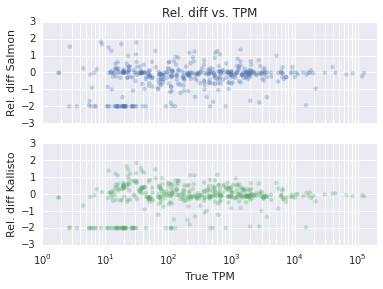# Now, with Counts¶

In :
rdK, nzK = relDiff("NumReads_kallisto", "NumReads_truth", truthKallisto)
medRelDiffKallisto = rdK["relDiff"].median()

medRelDiffSalmon = rdS["relDiff"].median()

In :
print("Kallisto")
print("===============================")
print("Median relative difference = {}".format(medRelDiffKallisto))
print("Median relative difference (non-zero) = {}".format(rdK["relDiff"][nzK].median()))
print("Mean relative difference = {}\n".format(rdK["relDiff"].mean()))

print("\n\nSalmon")
print("===============================")
print("Median relative difference = {}".format(medRelDiffSalmon))
print("Median relative difference (non-zero) = {}".format(rdS["relDiff"][nzS].median()))
print("Mean relative difference = {}\n".format(rdS["relDiff"].mean()))

Kallisto
===============================
Median relative difference = 0.0
Median relative difference (non-zero) = 0.00831900446451
Mean relative difference = 0.0288764285793

Salmon
===============================
Median relative difference = 0.0
Median relative difference (non-zero) = 0.00222828383726
Mean relative difference = 0.0333436111112


In :
p = plt.hist(np.clip(relDiffReads["relDiff_salmon"], -2.0, 2.0), bins=100)
_ = plt.axes().set_ylim((0,800))
_ = plt.axes().set_title("Salmon Reads Relative Difference")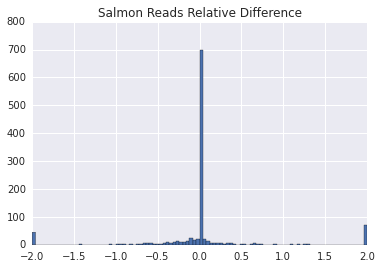In :
p = plt.hist(np.clip(relDiffReads["relDiff_kallisto"], -2.0, 2.0), bins=100)
_ = plt.axes().set_ylim((0,800))
_ = plt.axes().set_title("Kallisto Reads Relative Difference")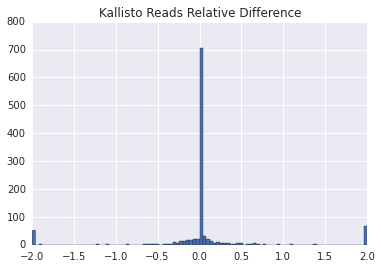In :
f, ax = plt.subplots(2, 1, sharex=True)